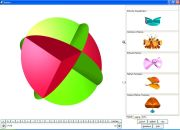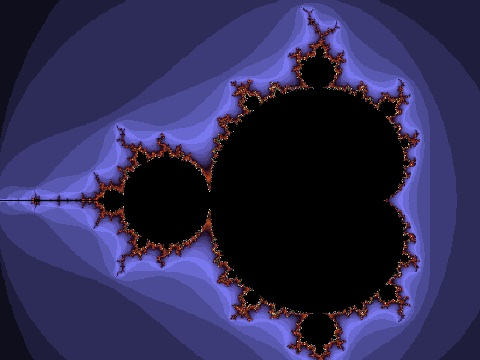## 24 Search Results

### Axiom

Axiom is a general purpose Computer Algebra system. It is useful for research and development of mathematical algorithms. It defines a strongly typed, mathematically correct type hierarchy. It has a programming language and a built-in compiler.

### GAP

GAP is a system for computational discrete algebra, with particular emphasis on Computational Group Theory. GAP provides a programming language, a library of thousands of functions implementing algebraic algorithms written in the GAP language as well as large data libraries of algebraic objects. GAP is used in research and teaching for studying groups and their representations, rings, vector spaces, algebras, combinatorial structures, and more. GAP is developed by international cooperation. The system, including source, is distributed freely under the terms of the GNU General Public License. You can study and easily modify or extend GAP for your special use. The current version is GAP 4, the older version GAP 3 is still available.

### Gauss

Introduction: Gauss is an easy-to-use data analysis, mathematical and statistical environment based on the powerful, fast and efficient GAUSS Matrix Programming Language. It is used to solve problems of exceptionally large scale. Program development and program execution are fast. Programs in command line mode (as in DOS or Unix); a limited Windows graphical user interface. GAUSS plot features a fully functional, interactive GUI. It can be used as a tool to design their own algorithms, for doing quick simulations, to write compact programs given the number of matrix-based statistical and financial functions, to used for numerical computation, and handle matrices in the same way as scalars. It provides a C-library interface.

### Global Optimization Toolbox For Maple

Optimization is the science of finding solutions that satisfy complicated constraints and objectives. In engineering, constraints may arise from technical issues. In business, constraints are related to many factors, including cost, time, and staff. The objective of global optimization is to find [numerically] the absolute best solution of highly nonlinear optimization models that may have a number of locally optimal solutions. Global optimization problems can be extremely difficult. Frequently engineers and researchers are forced to settle for solutions that are “good enough” at the expense of extra time, money, and resources, because the best solution has not been found. Using the Global Optimization Toolbox, you can formulate your optimization model easily inside the powerful Maple numeric and symbolic system, and then use world-class Maple numeric solvers to return the best answer, fast! Illustrative references: 1. Pintér, J. D. Global Optimization in Action. Springer Science, 1996, 512 p., ISBN: 978-0-7923-3757-7 Winner of the 2000 INFORMS Computing Society Prize. 2. Pintér, J. D., Linder, D. and Chin, P. Global Optimization Toolbox for Maple: An introduction with illustrative applications. Optimization Methods and Software 21 (2006) (4) 565-582.

### GOBLIN Graph Library

A C++ class library including the whole bunch of standard algorithms in graph optimization and drawing. On top of this, a Tcl/Tk wrapper and a GUI for manipulating and editing of graphs.

### IBM SPSS Statistics

With IBM SPSS Statistics, you can get a quick look at your data, formulate hypotheses for additional testing, and then carry out a number of procedures to help clarify relationships between variables, create clusters, identify trends and make predictions.

### igraph

igraph is a collection of network analysis tools with the emphasis on efficiency, portability and ease of use. igraph is a free and open source software package for creating and manipulating undirected and directed graphs. It includes implementations for classic graph theory problems like minimum spanning trees and network flow, and also implements algorithms for some recent network analysis methods, like community structure search. The efficient implementation of igraph allows it to handle graphs with millions of vertices and edges. The rule of thumb is that if your graph fits into the physical memory then igraph can handle it. igraph can be programmed in R, Python and C/C++ by virtue of R/igraph, python-igraph and C/igraph, respectively. There is also a Mathematica interface IGraph/M written by Szabolcs Horvát.

### ILOG CPLEX

ILOG CPLEX is an environment for optimization problems. ILOG CPLEX algorithms can be accessed from the CPLEX Component Libraries as well as the CPLEX Interactive Optimizer, an easy-to-use interactive program. CPLEX provides all the basic features and utilities for using these solvers: sophisticated problem preprocessing; file reading and writing utilities; reporting; messaging control; interactive revision capability; efficient restart from an advanced basis; sensitivity analysis; and an infeasibility finder.

### JMP

JMP provides a comprehensive set of statistical tools as well as design of experiments and statistical quality control in a single package. It allows for custom programming and script development via JSL, "JMP Scripting Language".

### JMulTi

JMulTi is an interactive software designed for univariate and multivariate time series analysis. It has a Java graphical user interface that uses an external engine for statistical computations. It has been designed for certain econometric procedures in time series analysis, especially some of them not available in other packages, like Impulse Response Analysis with bootstrapped confidence intervals for VAR/VEC modelling.

### Maple

Maple is an environment for scientific and engineering problem-solving, mathematical exploration, data visualization and technical authoring.

More information### Matlab

MATLAB is a high-level language and interactive environment that enables you to perform computationally intensive tasks faster than with traditional programming languages such as C, C++, and Fortran.

### Maxima

Maxima is a system for the manipulation of symbolic and numerical expressions, including differentiation, integration, Taylor series, Laplace transforms, ordinary differential equations, systems of linear equations, and vectors, matrices, and tensors. Maxima produces high precision results by using exact fractions and arbitrarily long floating point representations, and can plot functions and data in two and three dimensions.

### polymake

polymake is an object-oriented system for experimental discrete mathematics. The typical working cycle of a polymake user starts with the construction of an object of interest, auch as a convex polytope, a finite simplicial complex, a graph, etc. It is then possible to ask the system for some of the object's properties or for some form of visualization. Further steps might include more elaborate constructions based on previously defined objects. Each class of polymake objects comes with a set of rules which describe how a new property of an object can be derived from previously known ones. It is a key feature that the user can extend or modify the set of rules, add further properties or even new classes of objects (with entirely new rule bases). The functions provided include: several convex hull algorithms, face lattices of convex polytopes, Voronoi diagrams and Delaunay decompositions (in arbitrary dimensions), simplicial homology (with integer coefficients), simplicial cup and cap products, intersection forms of triangulated 4-manifolds. Several forms of (interactive) visualization via interfaces to Geomview, JavaView and other programs.

### RATS

RATS (Regression Analysis of Time Series) is a fast, efficient, and comprehensive econometrics and time series analysis software package.

### Reduce

REDUCE is an interactive system for general algebraic computations of interest to mathematicians, scientists and engineers. It has been produced by a collaborative effort involving many contributors. It is often used as an algebraic calculator for problems that are possible to do by hand. However, REDUCE is designed to support calculations that are not feasible by hand. Many such calculations take a significant time to set up and can run for minutes, hours or even days on the most powerful computers.

### Risa/Asir

Risa/Asir is a general computer algebra system and also a tool for various computation in mathematics and engineering. The development of Risa/Asir started in 1989 at FUJITSU. Binaries have been freely available since 1994 and now the source code is also free. Currently Kobe distribution is the most active branch of its development. We characterize Risa/Asir as follows: (1) An environment for large scale and efficient polynomial computation. (2) A platform for parallel and distributed computation based on OpenXM protocols.

### Sage

SAGE is a framework for number theory, algebra, and geometry computation. It is open source and freely available under the terms of the GNU General Public License (GPL). SAGE is a Python library with a customized interpreter. It is written in Python, C++, and C (via Pyrex). Python (http://www.python.org) is an open source object-oriented interpreted language, with a large number of libraries, e.g., for numerical analysis, which are available to users of SAGE. Python can also be accessed in library mode from C/C++ programs. SAGE provides an interface to several important open source libraries, including Cremona’s MWRANK library for computing with elliptic curves, the PARI library (pari.math.u-bordeaux.fr) for number theory, Shoup’s number theory library NTL (http://www.shoup.net/ntl/), SINGULAR (http://www.singular.uni-kl.de) for commutative algebra, GAP (http://www.gap-system.org) for group theory and combinatorics, and maxima (http://maxima.sourceforge.net) for symbolic computation and calculus.

More information### SOFA

SOFA - Statistics Open For All The user-friendly, open-source statistics, analysis, and reporting package.

### STATGRAPHICS Online

STATGRAPHICS Online is a statistical package that runs within a web browser. You can enter data directly into the data editor or read data from text files, Excel files, or other common formats. The calculations are performed remotely on a web server and the results returned to your browser as HTML with imbedded graphics images.

### STATISTICA

STATISTICA provides a comprehensive array of data analysis, data management, data visualization, and data mining procedures. Its techniques include a wide selection of predictive modeling, clustering, classification, and exploratory techniques in one software platform.

### Surfer

Surfer is a programme to visualize real algebraic surfaces in an intuitive and fast way

More information### SymPy

SymPy is a Python library for symbolic mathematics. It aims to become a full-featured computer algebra system (CAS) while keeping the code as simple as possible in order to be comprehensible and easily extensible. SymPy is written entirely in Python and does not require any external libraries.

More information